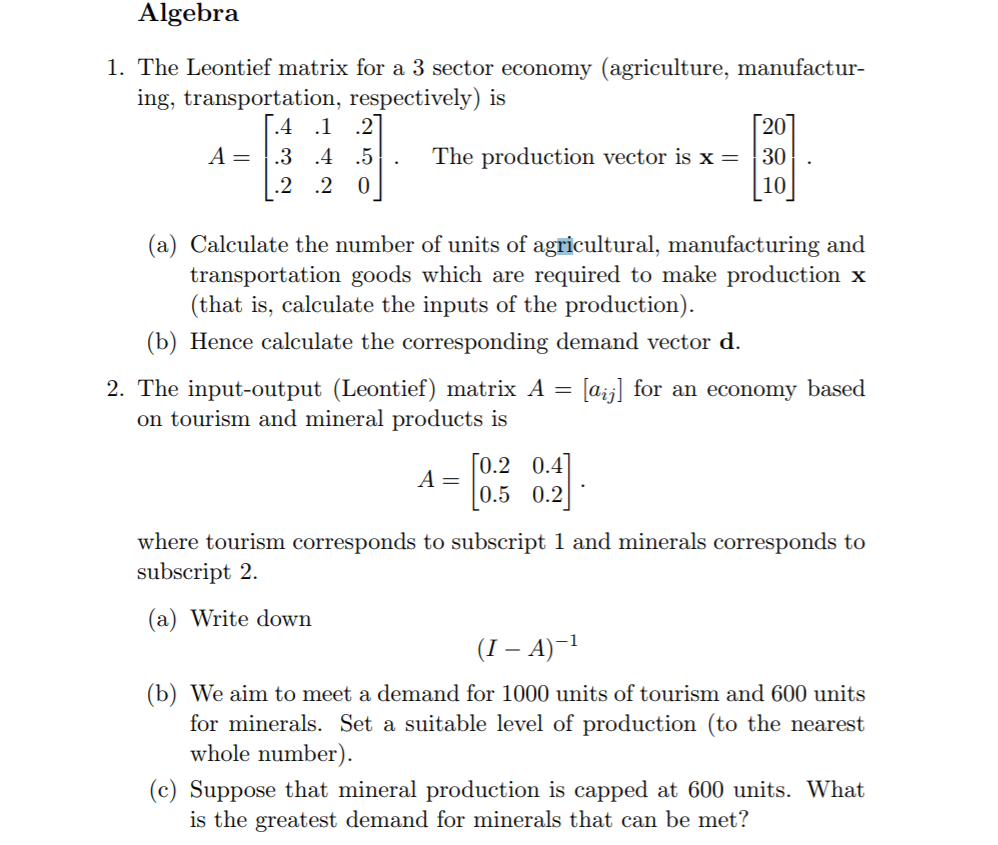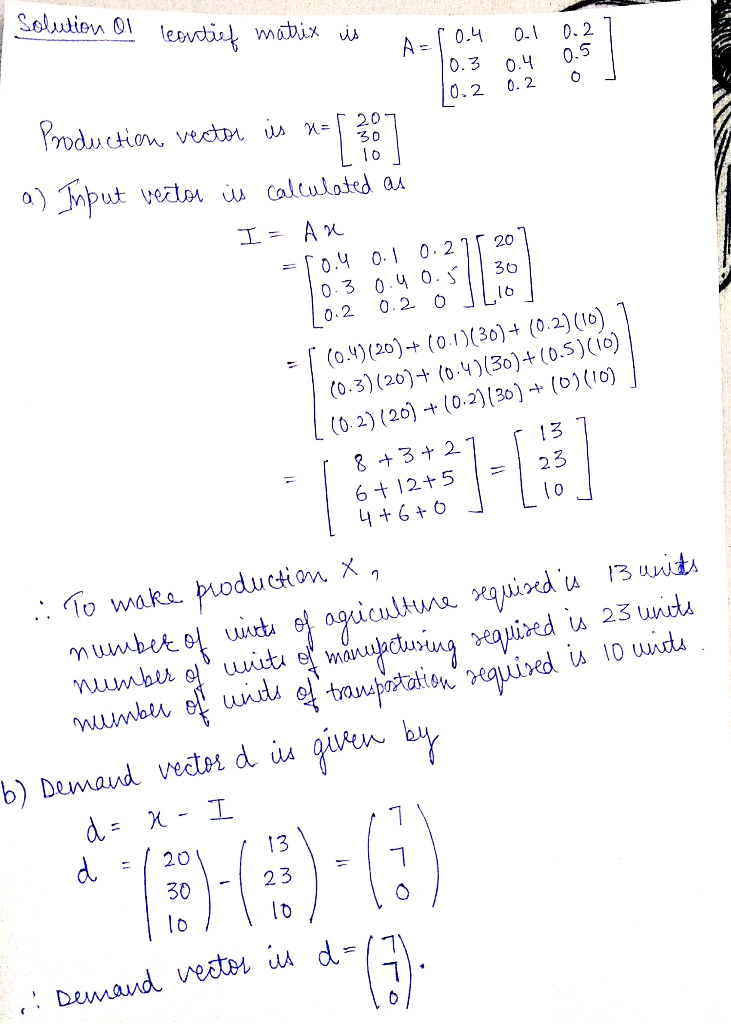Homework Help Question & Answers

Algebra 1. The Leontief matrix for a 3 sector economy (agriculture, manufactur ing, transportation, respectively) is 20 A=1.3 .4 .5 The production vector is x = 130 10 (a) Calculate the number of uni...Algebra 1. The Leontief matrix for a 3 sector economy (agriculture, manufactur ing, transportation, respectively) is 20 A=1.3 .4 .5 The production vector is x = 130 10 (a) Calculate the number of units of agricultural, manufacturing and transportation goods which are required to make production x (that is, calculate the inputs of the production) (b) Hence calculate the corresponding demand vector d. aij] for an economy based 2. The input-output (Leontief) matrix A on tourism and mineral products is 0.2 0.4 0.5 0.2 where tourism corresponds to subscript 1 and minerals corresponds to subscript 2 (a) Write down (I-A)-1 (b) We aim to meet a demand for 1000 units of tourism and 600 units for minerals. Set a suitable level of production (to the nearest whole number) (c) Suppose that mineral production is capped at 600 units. What is the greatest demand for minerals that can be met?Add Answer of: Algebra 1. The Leontief matrix for a 3 sector economy (agriculture, manufactur ing, transportation, respectively) is 20 A=1.3 .4 .5 The production vector is x = 130 10 (a) Calculate the number of uni...
More Homework Help Questions Additional questions in this topic.

• In Exercises 8-13 solve the given transportation problem. 70 50 30 100 8. C-19 6 2 1, s-| 90, and d- 130 In Exercises 8-13 solve the given transportation problem. 70 50 30 100 8. C-19 6 2 1, s-|...

Need Online Homework Help?

Get FREE EXPERT Answers
WITHIN MINUTES
Related Questions